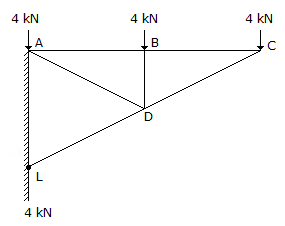# Civil Engineering - UPSC Civil Service Exam Questions

46.

A jet of water issues from a 5 cm diameter nozzle, held vertically upwards, at a velocity of 20 m/sec. If air resistance consumers 10% of the initial energy of the jet, then it would reach a height, above the nozzle, of

 A. 18.35 m B. 19.14 m C. 19.92 m D. 20.00 m

Explanation:

No answer description available for this question. Let us discuss.

47.

A given material has Young's modulus E, modulus of rigidity G and Poisson's ratio of Young's modulus to modulus of rigidity of this material is,

 A. 3.75 B. 3 C. 2.5 D. 1.5

Explanation:

No answer description available for this question. Let us discuss.

48.

The force in the member BD of the truss shown in the given figure isA. 4 kN tensile B. 4 kN compressive C. zero D. 12 kN compressive

Explanation:

No answer description available for this question. Let us discuss.

49.

Which of the following instruments have both horizon glass and index glass ?
1. Optical square
2. Line ranger
3. Box sextant
4. Pedometer
Select the correct answer using the code given below :

 A. 2, 3 and 4 B. 1, 3 and 4 C. 1 and 3 only D. 2 and 4 only

Explanation:

No answer description available for this question. Let us discuss.

50.

Which of the following equations are used for the derivation of the differential equation for water surface profile in open channel flow :
1. Continuity equation
2. Energy equation
3. Momentum equation
Select the correct answer using the codes given below :

 A. 1, 2 and 3 B. 1 and 3 C. 2 and 3 D. 1 and 2